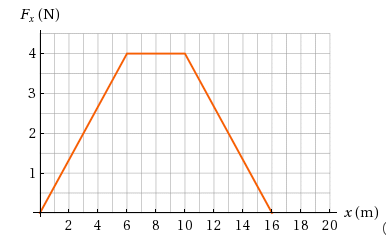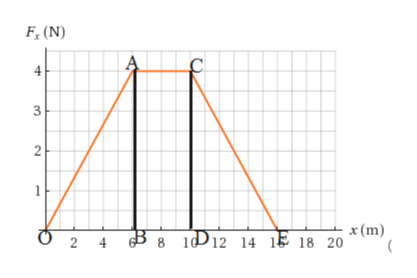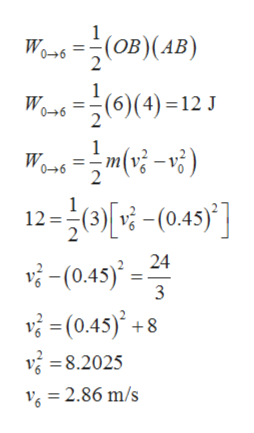Question
4 views

A object of mass 3.00 kg is subject to a force Fx that varies with position as in the figure below.

(d) If the object has a speed of 0.450 m/s at x = 0, find its speed at x = 6.00 m and its speed at x = 16.0 m.

speed at x = 6.00 m =  ?

speed at x = 16.0 m = ?help_outlineImage TranscriptioncloseF, (N) 4 3 2 x (m) 2 4 6. 8 10 12 14 16 18 20 fullscreen
check_circle

Step 1

Given,

Step 2

Area of graph F-x will is equal to work done.help_outlineImage TranscriptioncloseF, (N) 1 x (m) B 8 1D12 14 1Ệ 18 20 2 4 3. 2. fullscreen
Step 3

Work done between x= 0 to x= 6 m is equal to change in kinetic ...help_outlineImage TranscriptioncloseVons =(0B)(4B) 0-6 (6)(4) =12 J 0-6 Wons =m(v; -v}) 12=0[ri-(043"] v -(0.45) - 0-6 2 v -(0.45)* 24 3 v = (0.45)° +8 v = 8.2025 V = 2.86 m/s fullscreen

### Want to see the full answer?

See Solution

#### Want to see this answer and more?

Solutions are written by subject experts who are available 24/7. Questions are typically answered within 1 hour.*

See Solution
*Response times may vary by subject and question.
Tagged in

### Physics